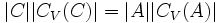# Timmesfeld's replacement theorem

Suppose$G$ is a finite group,$p$ is a prime number, and$V$ is a faithful$\mathbb{F}_p(G)$-module, i.e., a vector space over the field of$p$ equipped with a$G$-action. Consider the set$s_p(G,V)$ of elementary abelian$p$-subgroups$A$ of$G$ such that$|A||C_V(A)| \ge |B||C_V(B)|$ for all subgroups$B$ of$A$. Suppose$A \in s_p(G,V)$. Then, we have:
•$C = C_A([V,A])$ is nontrivial.
•$|C||C_V(C)| = |A||C_V(A)|$. In particular,$C \in s_p(G,V)$.
•$|C_V(C)| = [V,A]C_V(A)$.